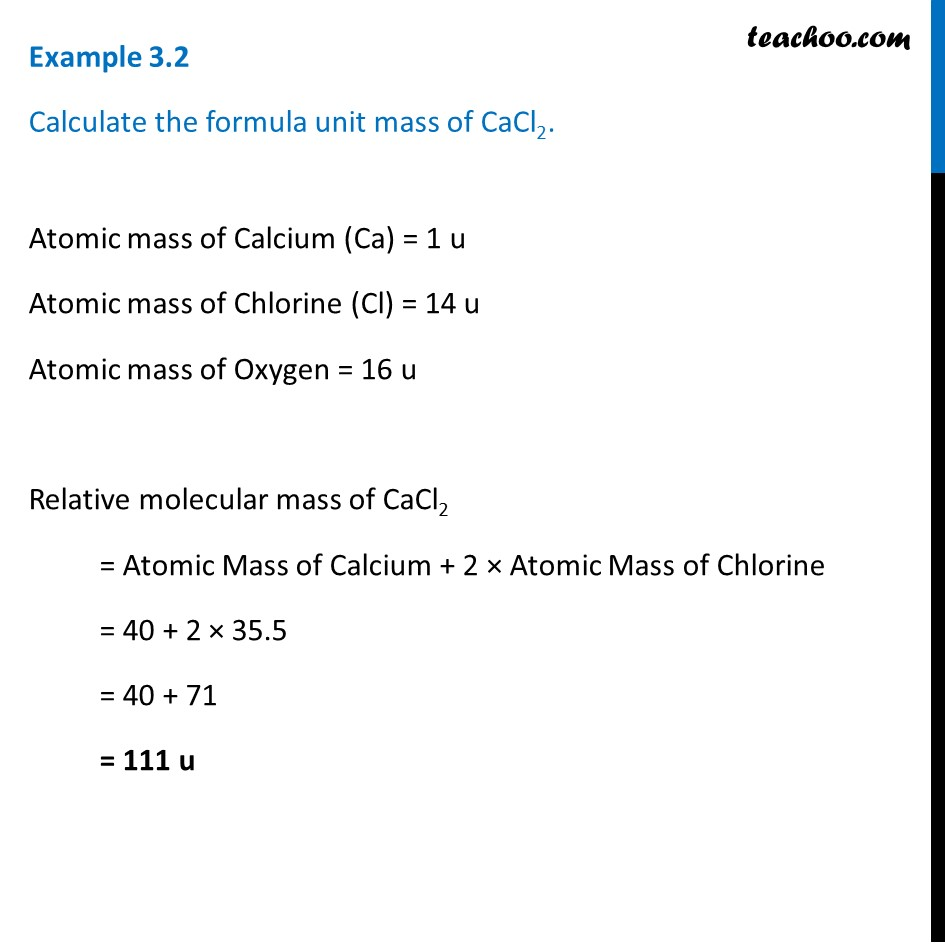1. Class 9
2. Chapter 3 Class 9 - Atoms And Molecules (Term 2)
3. Examples from NCERT Book

Transcript

Example 3.2 Calculate the formula unit mass of CaCl2. Atomic mass of Calcium (Ca) = 1 u Atomic mass of Chlorine (Cl) = 14 u Atomic mass of Oxygen = 16 u Relative molecular mass of CaCl2 = Atomic Mass of Calcium + 2 × Atomic Mass of Chlorine = 40 + 2 × 35.5 = 40 + 71 = 111 u

Examples from NCERT Book

Class 9
Chapter 3 Class 9 - Atoms And Molecules (Term 2)